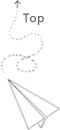# Caculating process layout

use the dp programming to caculate the process layout

In MPI cartisian, there is one useful interface (MPI_Dims_create) to caculate the number of the process (process layout) at each dimention according to the total process number.

this function is useful to caculte the size of the local domain according to the process number to make the program general. For example, the total mesh size is 128 times 128, and then the total process number is 4, the local mesh size is 64 times 64. the process layout is 2 times 2, which means each dimintion will be divided into 2 parts in order to make the total partition number equals to the 4 to let each rank associated with one particular domain.

The basic operation here is to caculate the number of the process in each dimention pi and make the p1 time p2 time … pn = total number of the process. This is a typical process for the searching based algorithm. Assume the total process number is p than we could divid it into the subproblem, the p = p1 time po, p1 is all the possibilities that is exact division by p. Then we use the similar strategy for the po, which is a subproblem, by this way, we can traverse all the possibilities.

this is a sample code to solve this problem

Basically, it use the DFS and the leaf node is the case when the dn is 1, which means remaining dimention is 1. We could print the results out:

Based on this framework, we may consider more, such as store all solutions into a data structure vector< vector <int> >, and to used by other solutions. This is how we use a vector to record the solution, it is important to consider when to push and when to pop, by this way, we could reuse the current vector during the process of the search. The similar strategies may used in lots of similar dynamic programming problems.

The results may looks more clear in this case:

For the next step, we may consider how to add more constrains and use the dynamic programming to store some intermediate status to solve the problem. For exmaple, we may want the difference between different number of in each dimention as small as possible.

The straightforward method is to get all solutions then check it based on the constrains. But we could also use some trim operations to cut the unnecessary iterations during the traverse, for example, if we set our goal as “find a solution that is most balanced one, which means the differences between value in each dimention is the minimal”, this is a typical process from the search based algorithm to the dynamica programming algoeithnm. For example, we could use a global variable to record the minimal differences and associated solution. If the differences of the current solution is larger than this value, we do not need to iterate further. otherwise, we will compute current solution recursively, if it’s difference is smaller than the minimal value, we replace it. There is tight relationship between the search based method and the dp based method (the setting is alwasy an optimization problem)

Inspired by this question, we could figure out the common strategy to solve the dp problem, the first step is to consider the traverse problem behind it. The second step is to consider what data structure need to be stored to reuse for solving the problem in a more large scale.

https://blog.csdn.net/runner668/article/details/79877458

## 推荐文章